# An Overview Of My R Shiny Math Tools Web Application

in STEMGeeks3 months ago

## An Overview Of My R Shiny Math Tools Web Application

Hello there. In this post, I would like to provide an overview of a math tools web application I have made in the R programming language along with the RShiny and the flexdashboard packages in R.

I won't be sharing code snippets as I prefer to showcase some features of this web application (with screenshots). There are free and cheap resources online for learning the R programming language for statistical work, data analysis, statistical modelling, hypothesis testing, etc. For learning RShiny I think a good place to start is this RShiny Tutorial from RStudio.

My math tools web application link is here.Pixabay Image Source

## Contents

• About The Math Tools Web Application
• Functions Plotting
• Geometry
• Personal Finance
• Quadratic Formula Calculator
• Future Works Ideas

## About The Math Tools Web Application

This web application in R & Shiny is a hobbyist side project I wanted to do. I wanted to combine mathematics with R programming into a sharable web application. Having something out there on the web does demonstrate some sort of proof of what I have learned from school, self-study along with trial and error.

A lot has put into this but it did not feel tiring. It is because I worked on this piece by piece over several weeks. I took a look a long break from this for a few months and completed the rest of this recently.

RShiny is nice as it allows for interactivity from the user. It does allow for widgets such as text boxes, colour lists, drop down select boxes and sliders. Being able to embed math text with the use of LaTeX (lay-tech) is nice for communicating mathematics. In addition, being able to include plotting with the use of R's ggplot2 package is very nice for graphing mathematical functions. The plots are not like Desmos or anything but it does a decent job.

There are a lot of blocks, squares and rectangular boxes. This is mainly from the flexdashboard package in R & RShiny. I could add more colour.

## Functions Plotting

When you open the web application link, the first thing you see is this: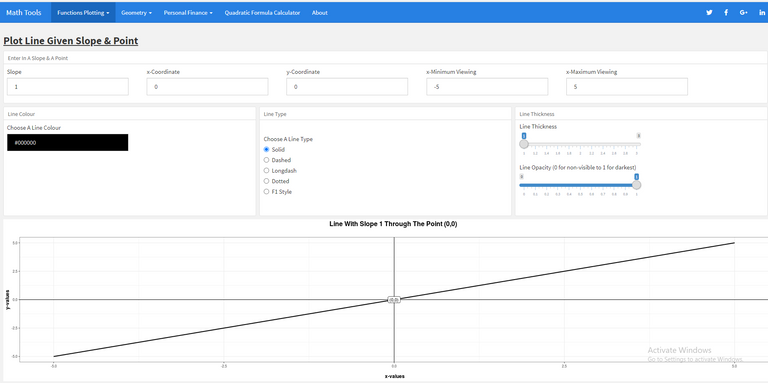The default home page is the Plot Line Given Slope & Point from the first section named Functions Plotting. You can change the slope of the line along with the (x, y) co-ordinate that the line passes through. Optional features include changing how the line looks through the line type, line colour, line thickness, and line opacity (transparency).

Polynomial Curve

The second tool under Functions plotting is the polynomial function plot. In this one, the user can plot a polynomial curve of up to the fifth degree. Simply input the co-efficient values in the polynomial and the ggplot2 graph from R will appear below. Just like with the line through a point graph, you can change how the curve looks with colour, line type, line thickness and opacity.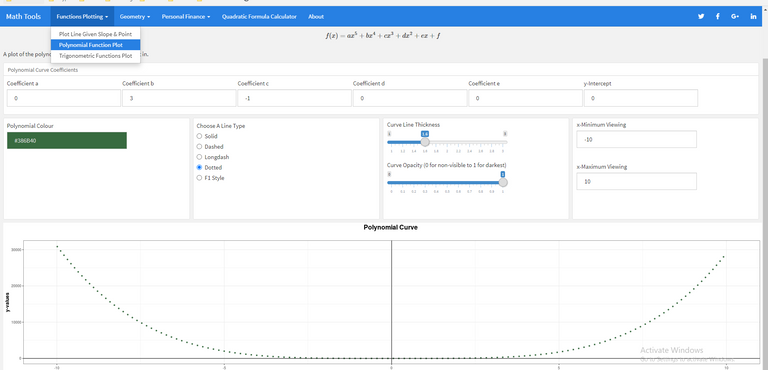Trigonometric Functions Plotter

The third tool from the Functions Plotting section is a plotter for the sine, cosine and tangent trigonometric functions. Users can change the vertical stretch of the trigonometric function, along with adjusting the horizontal phase shift, vertical shift and the period (one full cycle) in degrees.

A single trigonometric plot is shown below which adjusts to the user settings. This plotting tool in particular has tabs which allows for seeing a sine function plot, a cosine function plot or a tangent function plot based on the settings above the plot.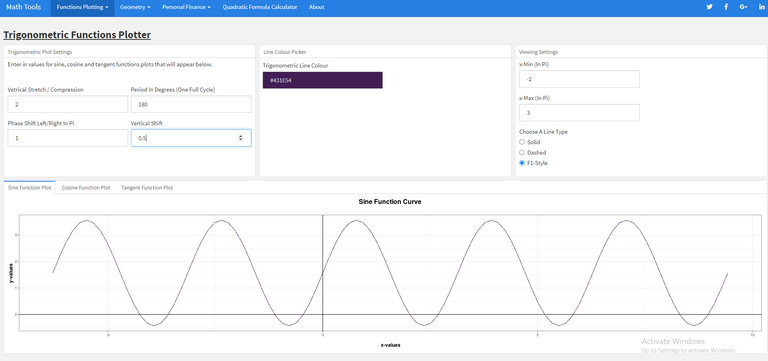## Geometry

In the second section, I have the geometry section. The geometry section is filled with calculators for computing perimeter and area of two dimensional shapes. I also have surface area and volume calculators for 3D shapes.

With the calculators, I have embedded LaTeX math text for the formulas. Here are a few screenshots.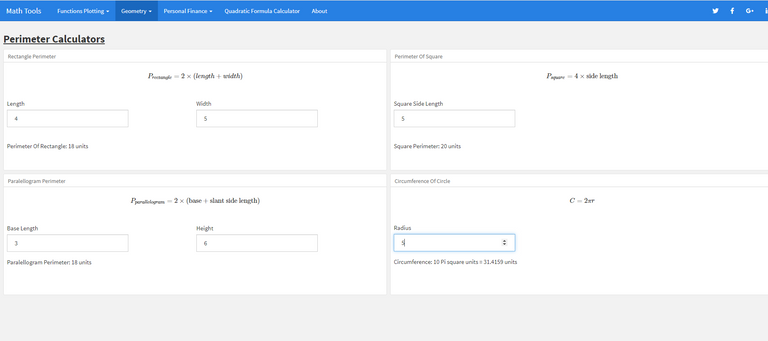Perimeter Calculators Page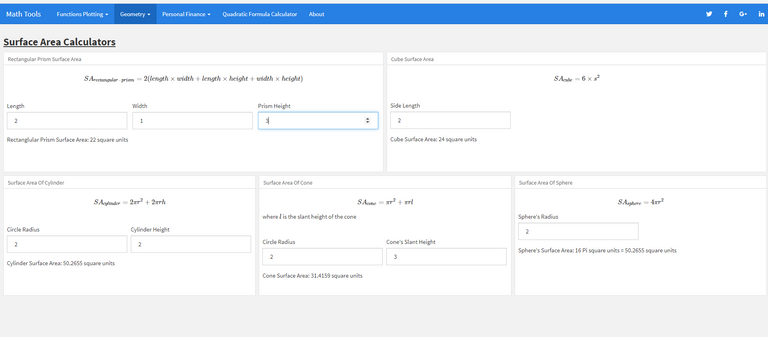Surface Area Calculators Page

## Personal Finance

In the personal finance section I have included two calculator types. The first part features two calculator converters while the second part is a compound interest calculator.

Annual vs Weekly Vs Hourly Wages Calculator

In the first converter the user can convert an hourly wage with number of hours per week and the number of working weeks in a year to obtain equivalent incomes on a monthly and annual basis.

The second calculator converter works in the reverse direction where the user inputs an annual salary and sets the number of working hours in a week and the number of working weeks in a year. From the inputs, the output for the second converter includes an equivalent hourly wage, an equivalent monthly income and an equivalent weekly salary.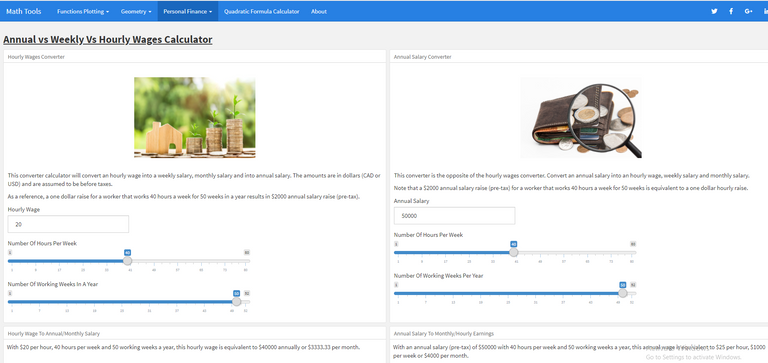Compound Interest Calculator

This calculator takes a principal amount for savings or a loan and applies compound interest to output a future value of the savings/loan. Users can input the principal amount, the interest rate, the number of years and type of interest rate compound.

I have included some mathematics on the left side for the exponential function compound interest formula.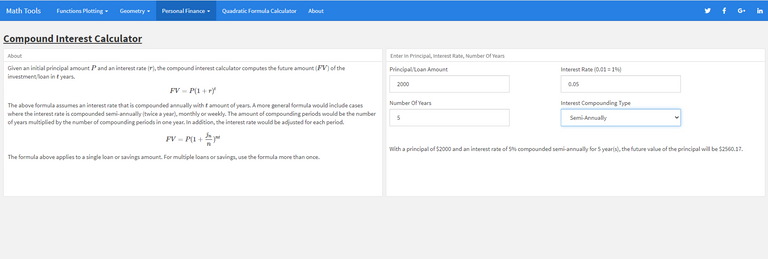## Quadratic Formula Calculator

The last part I would like to feature is the quadratic formula calculator. Input the coefficients for the quadratic equation and the quadratic calculator will output a result or a solution(s).

I have used if statements which outputs different results depending on the input values and the discriminant from the quadratic formula.

$$\text{If } b^2 - 4ac < 0 \implies \text{ No Real Valued Solutions (Negative Under Square Root)}$$
$$\text{If } b^2 - 4ac = 0 \implies \text{ One Root (Double Root)}$$
$$\text{If } b^2 - 4ac > 0 \implies \text{ Two Unique Solutions/Roots}$$

In addition to the quadratic formula calculator, I have added a basic quadratic function plot based on the quadratic coefficients inputs. I thought to myself, why not put it in. The plot also helps in terms of visualizing where the roots are what the quadratic function looks like and if it opens up or down.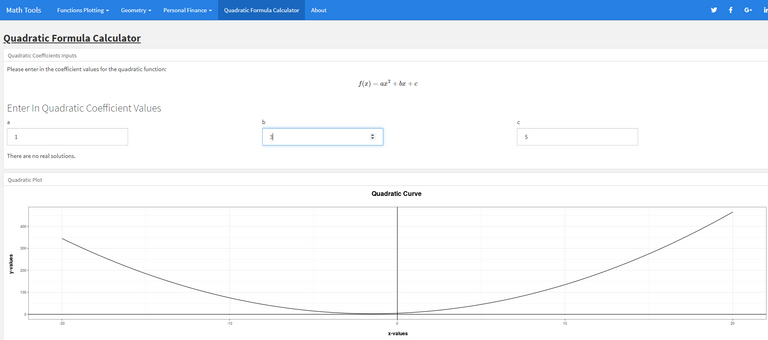Upwards Facing Parabola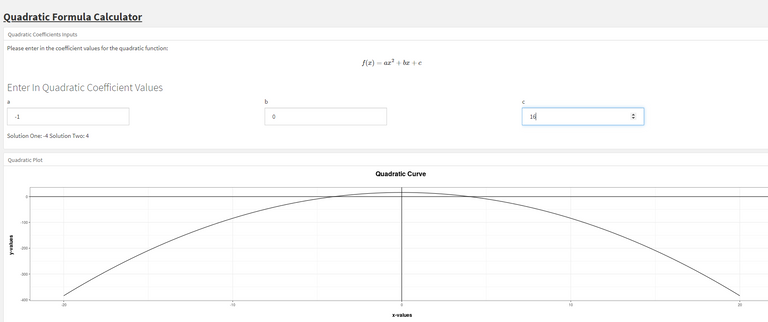Downwards Facing Parabola

## Future Works Ideas

I can still improve on it and even add things to it. There are two ideas I have in mind when it comes to adding on to this. One idea is for math flashcards. The math flashcards can be basic with the +, −, ×, and ÷ signs. I could do negative addition flashcards along with exponents flashcards. My second idea was a currency exchange converter tool.

There is also fixing any errors in this application. I spotted a few as I was testing some stuff and while typing this post out.Pixabay Image Source

Thank you for reading.

Posted with STEMGeeks

Sort:

Looks very neat and impressive. so what are the plans moving forward with the application you developed. cheers

I have a Foreign Exchange tool as an add-on to this. The post on this will arrive soon.

As another add-on I do plan on adding flashcards for addition, subtraction, multiplication and division. And possibly flashcards for exponents.